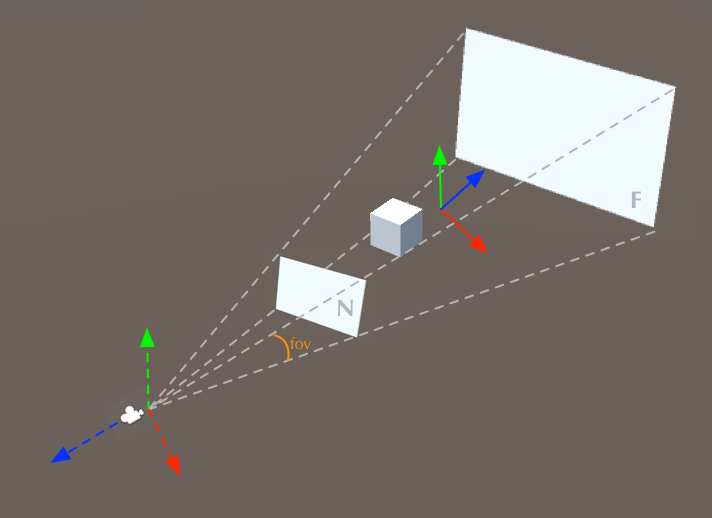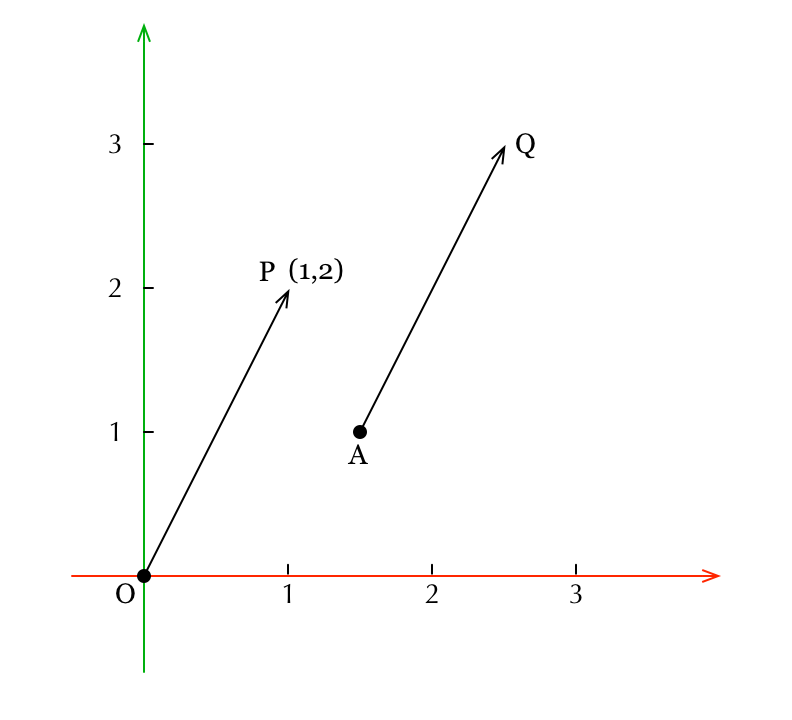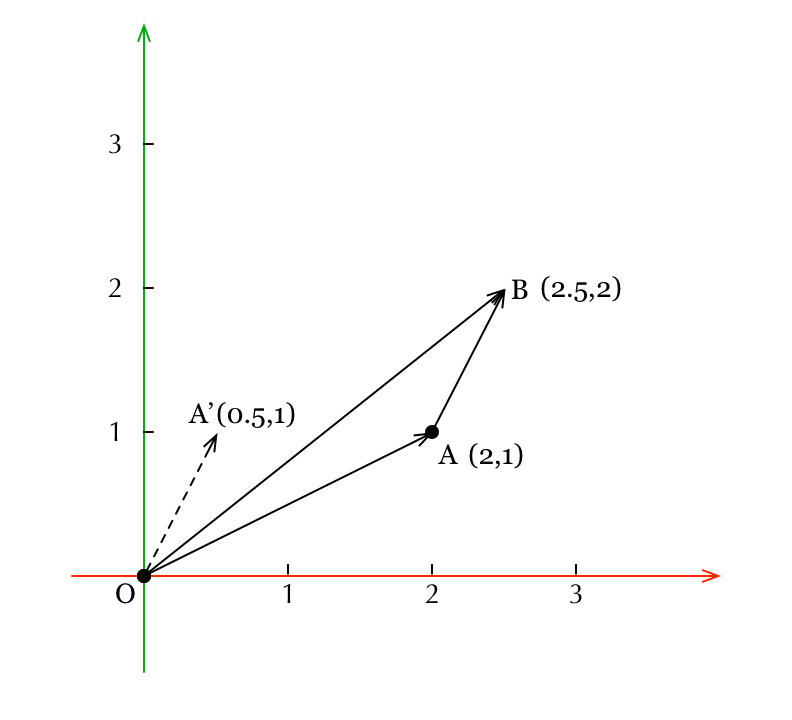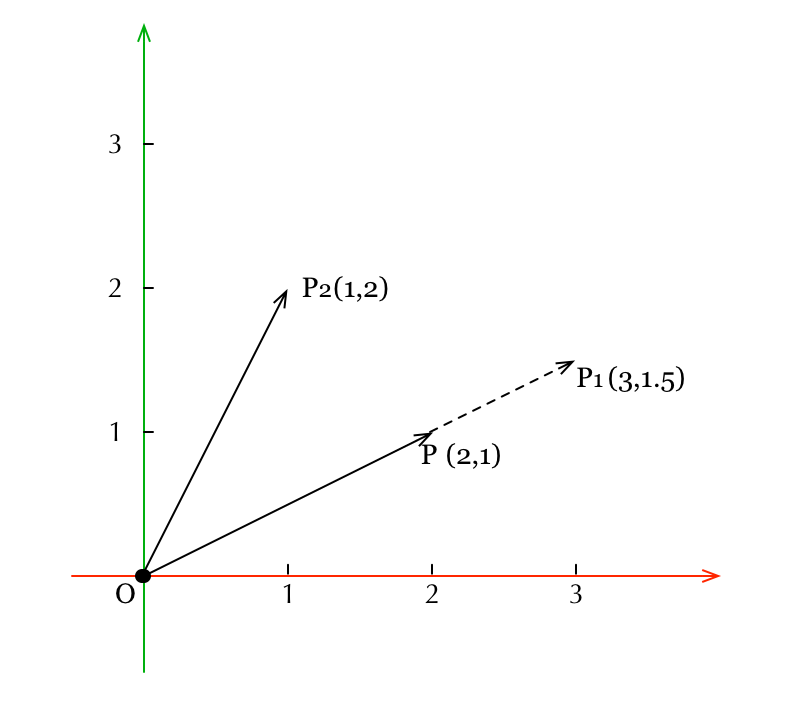## OpenGL ES和坐标变换（二） 2017-11-09

1. 首先，以Demo程序中的代码为例，介绍model、view、projection三个变换在Android中如何用代码来表达。
2. 介绍相关理论，推导一下model变换中的缩放(scaling)和平移(translation)这两个变换的计算过程。由于旋转(rotation)这个话题比较复杂，我们留在下一篇再讨论。
3. 我们分析一下Android中的缩放(scaling)和平移(translation)变换的具体实现，将前面的理论推导同代码实现相互印证，以加深理解。

### Demo程序简介

gl_Position = projection * view * model * vec4(position.xyz, 1);


Matrix.setIdentityM(modelMatrix2, 0);
Matrix.translateM(modelMatrix2, 0, 0.5f, 1.0f, -1.5f);
Matrix.rotateM(modelMatrix2, 0, angle, 0.0f, 1.0f, 0.0f);
Matrix.scaleM(modelMatrix2, 0, 1.5f, 1.5f, 1.5f);


1. 缩放：先在x, y, z三个坐标轴上都放大1.5倍；
2. 旋转：再绕着向量[0.0, 1.0, 0.0]T(也就是y轴)旋转角度angle
3. 平移：沿x轴正向平移0.5个单位，沿y轴正向平移1.0个单位，沿z轴负向平移1.5个单位。Matrix.setLookAtM(viewMatrix, 0, 3.0f, 3.0f, 10.0f, 0.0f, 0.0f, 0.0f, 0.0f, 1.0f, 0.0f);


Matrix.perspectiveM(projectionMatrix, 0, 45.0f, width / (float) height, 0.1f, 100.0f);


• 观察视角为45.0度。这个值通常被称为field of view，简称fov。它的含义已在下图中标出。• 第二个参数为宽高比，指的是近平面(N)的宽高比。
• 第三和第四个参数分别表示近平面(N)和远平面(F)与相机的距离。

### 缩放和平移矩阵的推导

#### 关于线性代数理论基础的说明#### 平移矩阵的推导过程#### 缩放矩阵的推导过程### Android中缩放和平移的实现

Matrix.setIdentityM(modelMatrix2, 0);
Matrix.translateM(modelMatrix2, 0, 0.5f, 1.0f, -1.5f);
Matrix.rotateM(modelMatrix2, 0, angle, 0.0f, 1.0f, 0.0f);
Matrix.scaleM(modelMatrix2, 0, 1.5f, 1.5f, 1.5f);


public static void scaleM(float[] m, int mOffset,
float x, float y, float z);


M’ = M S

    public static void scaleM(float[] m, int mOffset,
float x, float y, float z) {
for (int i=0 ; i<4 ; i++) {
int mi = mOffset + i;
m[     mi] *= x;
m[ 4 + mi] *= y;
m[ 8 + mi] *= z;
}
}


  m[offset +  0] m[offset +  4] m[offset +  8] m[offset + 12]
m[offset +  1] m[offset +  5] m[offset +  9] m[offset + 13]
m[offset +  2] m[offset +  6] m[offset + 10] m[offset + 14]
m[offset +  3] m[offset +  7] m[offset + 11] m[offset + 15]


    public static void translateM(
float[] m, int mOffset,
float x, float y, float z) {
for (int i=0 ; i<4 ; i++) {
int mi = mOffset + i;
m[12 + mi] += m[mi] * x + m[4 + mi] * y + m[8 + mi] * z;
}
}


（完）